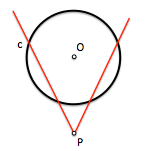# Metric geometry : Determination of lines with angular conditionsThe determination of a line in the plane requires two geometric constraints; among the employed conditions are the pass or membership of a point and angular type (form an angle with another line or circle).

Discuss the angular relation of a given condition to provide a method of obtaining solutions for reducing problems tangency circumference, valid for one or two angular conditions.

Consider the following problem:

Given a circle c center O The radio dado, and a point P external to the same, determine the lines through that point and form a given angle to the circumference.

In our problem the angle is a really problem, e.g. 45.

We have seen, to study the notions of angles, that the angle between a line and a circle is formed by the tangent line to the circle at the intersection point between both.

If the point P he was on the circumference (T), the solution would be immediate. We would get the tangent at T and then, with the value of the angle, would determine the direction of the line (r). The cutoff point of the line with the circle would be the point itself P=T.

If we turn the line with the center of the circle (O), the angle between the rotated line and the circle does not change. The infinite positions of this line, round, which are tangent to a circumference g Concentric to the previous c. This girth (g) called goniómetra.

We can change the angle of the line relative condition of the circumference c, by a tangency condition to the circumference goniómetra g.

To resolve the problem therefore first determine the circumference with angular goniómetra condition, and obtain the tangents to it from the point P. Need a arc capable of 90 º between the center O Common to the circumferences and the point P, to determine the points of contact in g.

Points I1 and I2 of tangency to goniómetra will be the crossing points of the solutions sought.

The circumference goniómetra therefore allows us to change geometric angularity condition other tangent that can apply in solving similar problems.

As an exercise for the reader intends to identify certain lines forming angles with two different circumferences, or an angle with a line and another with a circumference simultaneously.

Metric geometry